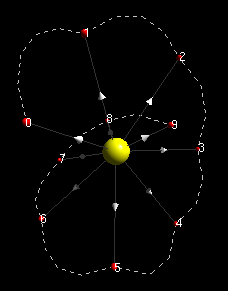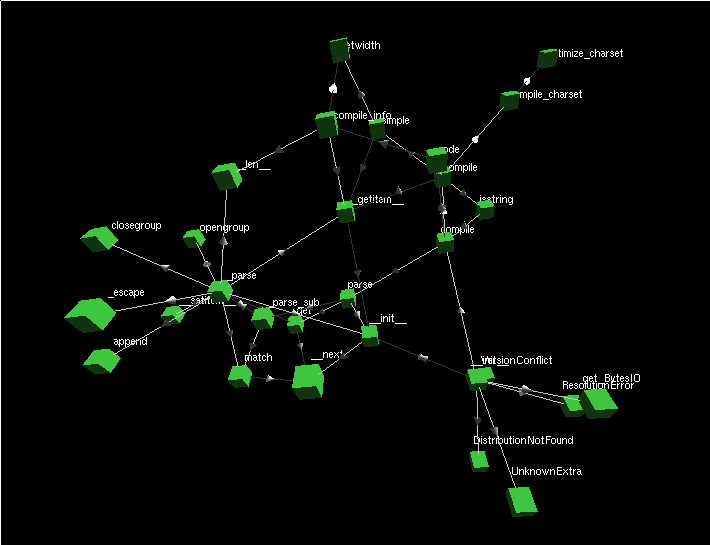2012-09-27

## UbiGraph 显示环境

UbiGraph是一个显示平台，可以非常方便地使用 Python/C/Ruby 来控制渲染，只需要制定节点和边还有其他相关属性，其余的都不用管了。其使用 XML-RPC 服务于客户端，所以甚至可以在一台机器上开 server，在另外一台机器上用渲染代码控制，这个环境对于算法和数据的可视化很有用。比如：

``````import ubigraph

U = ubigraph.Ubigraph()
U.clear()

x = U.newVertex(shape="sphere", color="#ffff00")

smallRed = U.newVertexStyle(shape="sphere", color="#ff0000", size="0.2")

previous_r = None
for i in range(0,10):
r = U.newVertex(style=smallRed, label=str(i))
U.newEdge(x,r,arrow=True)
if previous_r != None:
U.newEdge(r,previous_r,spline=True,stroke="dashed")
previous_r = r``````ubigraph_python

## 使用 Ubigraph 显示 Python 函数调用

``````import prof3d

def run_main():ubigraph_python